# Tree

(diff) ← Older revision | Latest revision (diff) | Newer revision → (diff)

in graph theory

A connected non-oriented graphwhich does not contain cycles. A tree has no multiple edges and no loops. Since they are the simplest possible, connected graphs, trees are suitable models for the study of various problems in graph theory. Any tree withvertices contains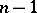edges. The number of different trees which may be constructed onnumbered vertices is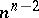. A tree with one distinguished vertex is said to be a rooted tree.

The enumerating seriesfor the number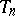of non-isomorphic rooted trees withvertices satisfies the functional equation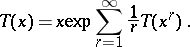The enumerating series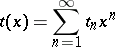for the number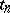of non-isomorphic trees withvertices can be represented using the enumerating series for rooted trees: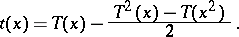The functional equations for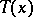and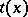yield the values ofandfor specific values of(see, for example, ).

It has been shown that, as,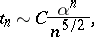where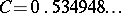,. Problems of enumeration of trees of a specific kind arise, for example, in chemistry in the study of isomers.

Trees may be fairly simply coded by strings of zeros and ones. Consider, for example, some regular (without intersection of edges) imbedding of a treein the plane (cf. Graph imbedding). Starting from any vertex, one moves along the edges of, turning at each vertex towards the first edge on the right, and returning in the terminal vertices of the tree. While moving along some edge, one writes 0 if the edge is traversed for the first time, and writes 1 when the edge is traversed for the second time (in the opposite direction). Ifis the number of tree edges, one returns to the starting vertex aftersteps, having passed each edge twice. The sequence of 0's and 1's of lengththus obtained (the code of the tree) makes it possible to uniquely reconstruct not only the treeitself, but also its imbedding in the plane. Several different codes correspond to one specific tree. The following estimate follows from this mode of coding:.

A treewithvertices can be uniquely retrieved (up to an isomorphism) from the set of all its-vertex subgraphs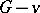, which are obtained fromby elimination of one of its vertices.

Any tree is also uniquely defined by the distances (length of the shortest path) between its terminal (valency 1) vertices.

A spanning tree of a graph is a subgraph of the given graph which contains all its vertices and which is a tree. The number of spanning trees of any given connected graphwithout loops and without multiple edges can be computed as follows. Letbe the matrix obtained from the adjacency matrix ofby replacing all the signs of the elements by the opposite signs and by replacing the-th entry on the principal diagonal by the valency of the vertex. The cofactors of all entries on the principal diagonal ofwill then all be equal, and their common value is the number of spanning trees of. Spanning trees are employed, for example, to find independent cycles in an electric circuit.

Of importance in applications is the problem of finding the spanning tree with the smallest sum of the edge-weights in a connected graph in which weights are assigned to the edges. Such a problem arises, for example, in designing communication networks. An algorithm for the solution of this shortest spanning tree problem is known , . A tree growing (or issuing) from a vertex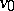is the name given to an oriented graph which is (disregarding the orientation) a rooted tree with root, and in which for any vertexthe (unique) chain connectingto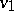is an oriented path fromto. Such trees are employed, for example, in the description of deterministic functions, for the representation of information in information-searching systems, etc.

The concept of a "forest" is a generalization of the concept of a "tree" . A forest is a graph without cycles (not necessarily connected).

How to Cite This Entry:
Tree. Encyclopedia of Mathematics. URL: http://encyclopediaofmath.org/index.php?title=Tree&oldid=16337
This article was adapted from an original article by V.B. Alekseev (originator), which appeared in Encyclopedia of Mathematics - ISBN 1402006098. See original article